# Wilcox Solar Observatory Magnetograms

## #8200 - 8209, 2005.01.23 - 2005.02.03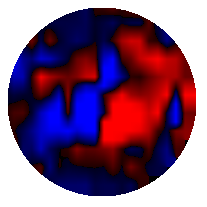```WSO Magnetogram sn=8200	 observed at 2005:01:23_21h:52m:44s
Central Meridian Carrington Longitude CT2025:072
Sky = 1 (5 worst)
trouble=00004000 NOTE:
12 intensity variance exceeds 5.0
``````WSO Magnetogram sn=8201	 observed at 2005:01:24_22h:38m:31s
Central Meridian Carrington Longitude CT2025:059
Sky = 2 (5 worst)
trouble=00004000 NOTE:
12 intensity variance exceeds 5.0
``````WSO Magnetogram sn=8202	 observed at 2005:01:26_22h:05m:56s
Central Meridian Carrington Longitude CT2025:033
Sky = 1 (5 worst)
trouble=00004000 NOTE:
12 intensity variance exceeds 5.0
``````WSO Magnetogram sn=8203	 observed at 2005:01:29_21h:02m:28s
Central Meridian Carrington Longitude CT2026:354
Sky = 0 (5 worst)
trouble=00004000 NOTE:
12 intensity variance exceeds 5.0
```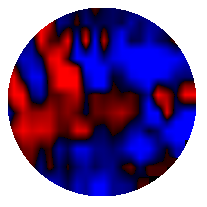```WSO Magnetogram sn=8204	 observed at 2005:01:30_22h:34m:30s
Central Meridian Carrington Longitude CT2026:340
Sky = 4 (5 worst)
trouble=00004000 NOTE:
12 intensity variance exceeds 5.0
```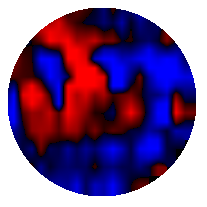```WSO Magnetogram sn=8205	 observed at 2005:01:31_21h:02m:56s
Central Meridian Carrington Longitude CT2026:327
Sky = 0 (5 worst)
trouble=00004000 NOTE:
12 intensity variance exceeds 5.0
```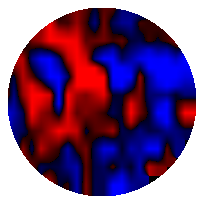```WSO Magnetogram sn=8206	 observed at 2005:01:31_23h:10m:50s
Central Meridian Carrington Longitude CT2026:326
Sky = 2 (5 worst)
trouble=00004000 NOTE:
12 intensity variance exceeds 5.0
```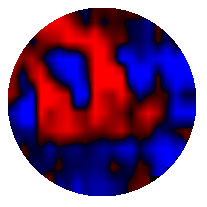```WSO Magnetogram sn=8207	 observed at 2005:02:01_21h:40m:40s
Central Meridian Carrington Longitude CT2026:314
Sky = 0 (5 worst)
trouble=00004000 NOTE:
12 intensity variance exceeds 5.0
```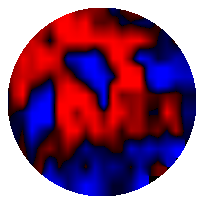```WSO Magnetogram sn=8208	 observed at 2005:02:02_22h:09m:43s
Central Meridian Carrington Longitude CT2026:300
Sky = 0 (5 worst)
trouble=00004000 NOTE:
12 intensity variance exceeds 5.0
```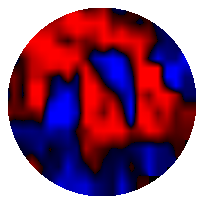```WSO Magnetogram sn=8209	 observed at 2005:02:03_22h:22m:36s
Central Meridian Carrington Longitude CT2026:287
Sky = 0 (5 worst)
trouble=00004000 NOTE:
12 intensity variance exceeds 5.0
```